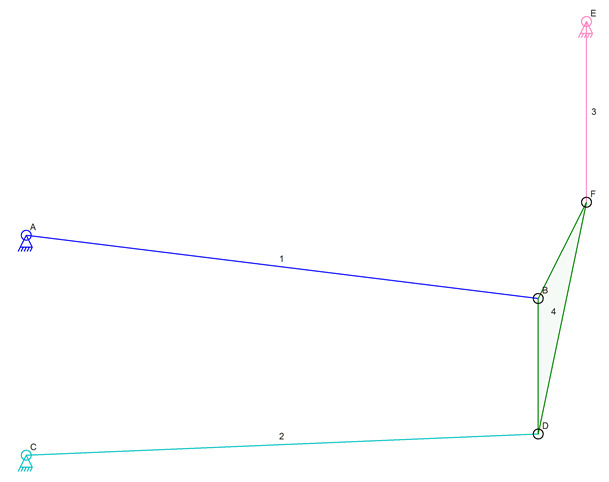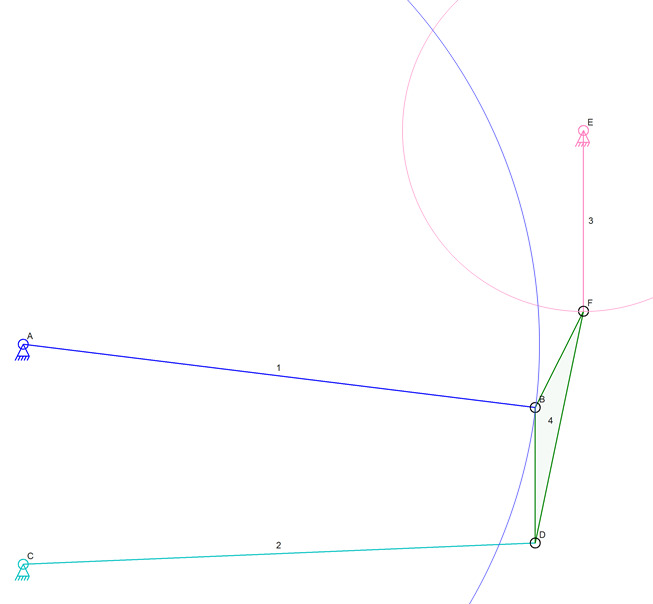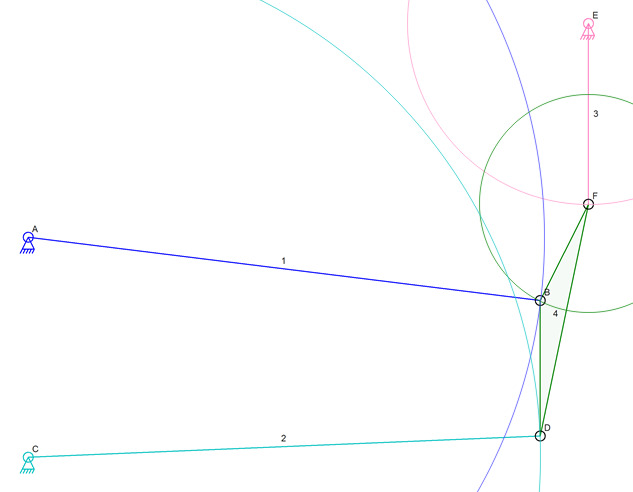I think that I am getting closer and closer to having a useable solution to the link positioning problem (blog post 1, blog post 2 ).

This new idea is based mostly on the last one. The idea was to use points on circles to narrow down the valid positions of the triangle link.

For review, here is a picture of a mechanism that cannot be simulated with the Linkage program:##### Part of Stephenson Valve Gear

This cannot be simulated because it has three connections to the triangle link that all define its location.

That’s enough review.

My latest idea is to take the circles formed around A and E by connectors B and F and to then find locations on them where the B and F connectors might be placed.The idea will be to start at a given location on circle E and move along the circle in small steps. At each position, a circle F1 will be calculated with a radius to match the distance from F to B. Where this circle intersects circle A is where connector B might be placed in relation to F1. With those two points, it is easy to find a location where D might be placed and that location can be checked to see if it is on the circle around C of radius CD.

A picture of the final resting place of the triangle link will show the various circle intersections that were used to find the points.##### Final Position

Point F was found on circle E by taking many iterations to get there. When the green circle around F was checked, it was determined that it intersected the blue circle A at two places. Assuming that one place didn’t yield useful results, the intersection at B is checked. This is where the idea differs from the previous idea; D is now calculated based on B and F and then checked to see if it is the correct distance from C. The distance calculation will be quicker than the circle to circle intersection test followed by other distance or angle tests that I thought of in the previous post blog .

With any of the iteration methods, I will need to do some results management so that I can deal with the fact that these are approximations and that D will never be the exact distance that is needed. I will need to find where during the stepping it goes from too close to too far and then do the same algorithm on that small section of the data using smaller steps. If it gets close then moves away again then the mechanism is too close to binding and might not simulate. Small allowances for that might make a binding simulation run but that would be better than having a good mechanism fail.

I think that some amount of variance in the number will be acceptable since there is no facility to detect binding in things like piston or valve mechanisms anyhow.